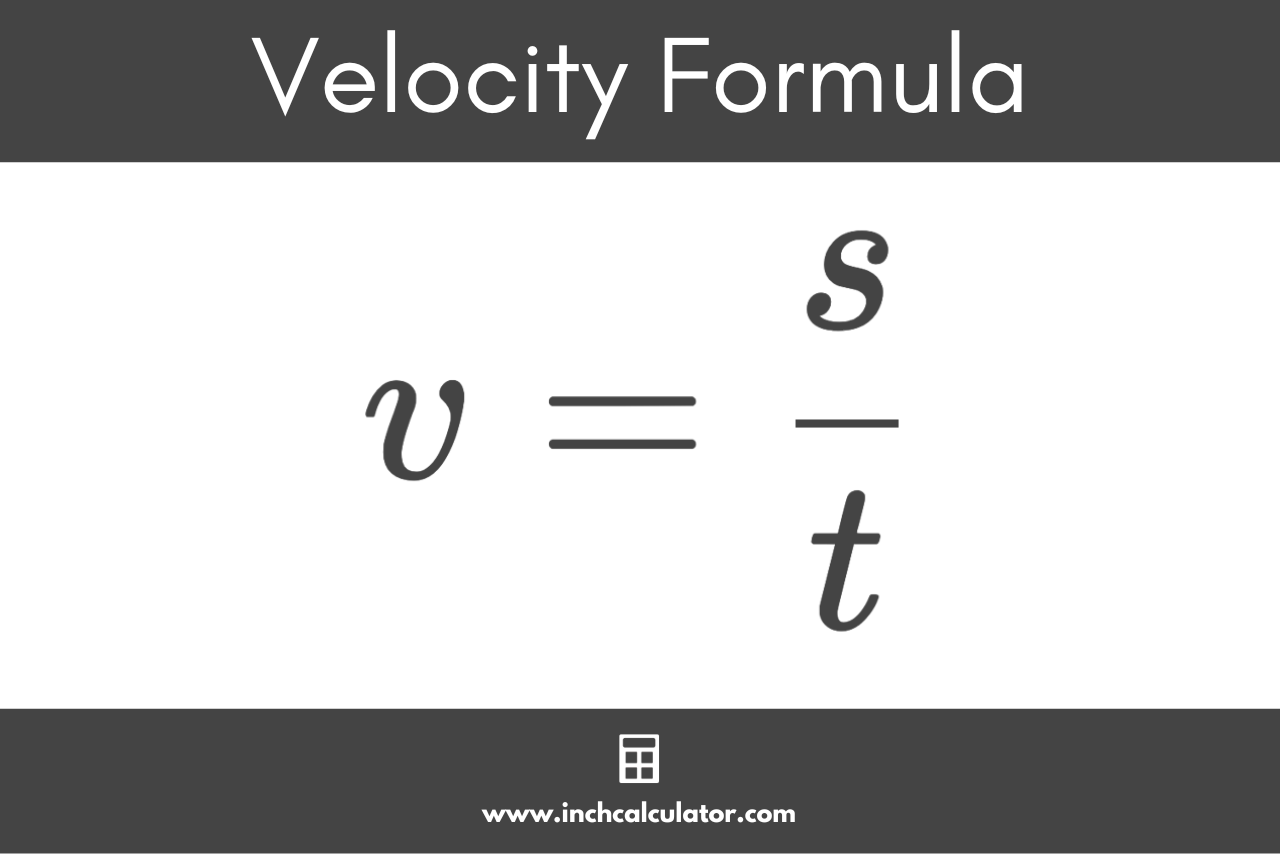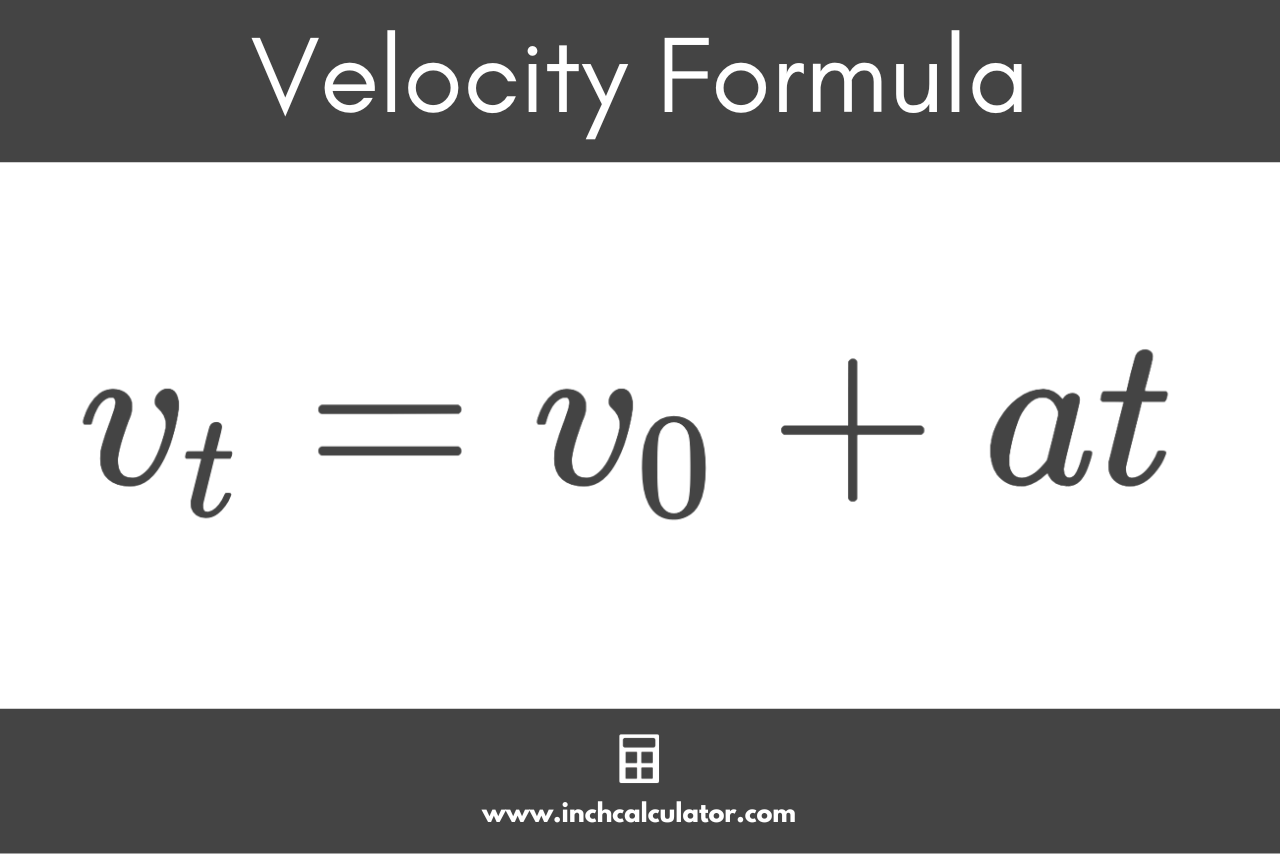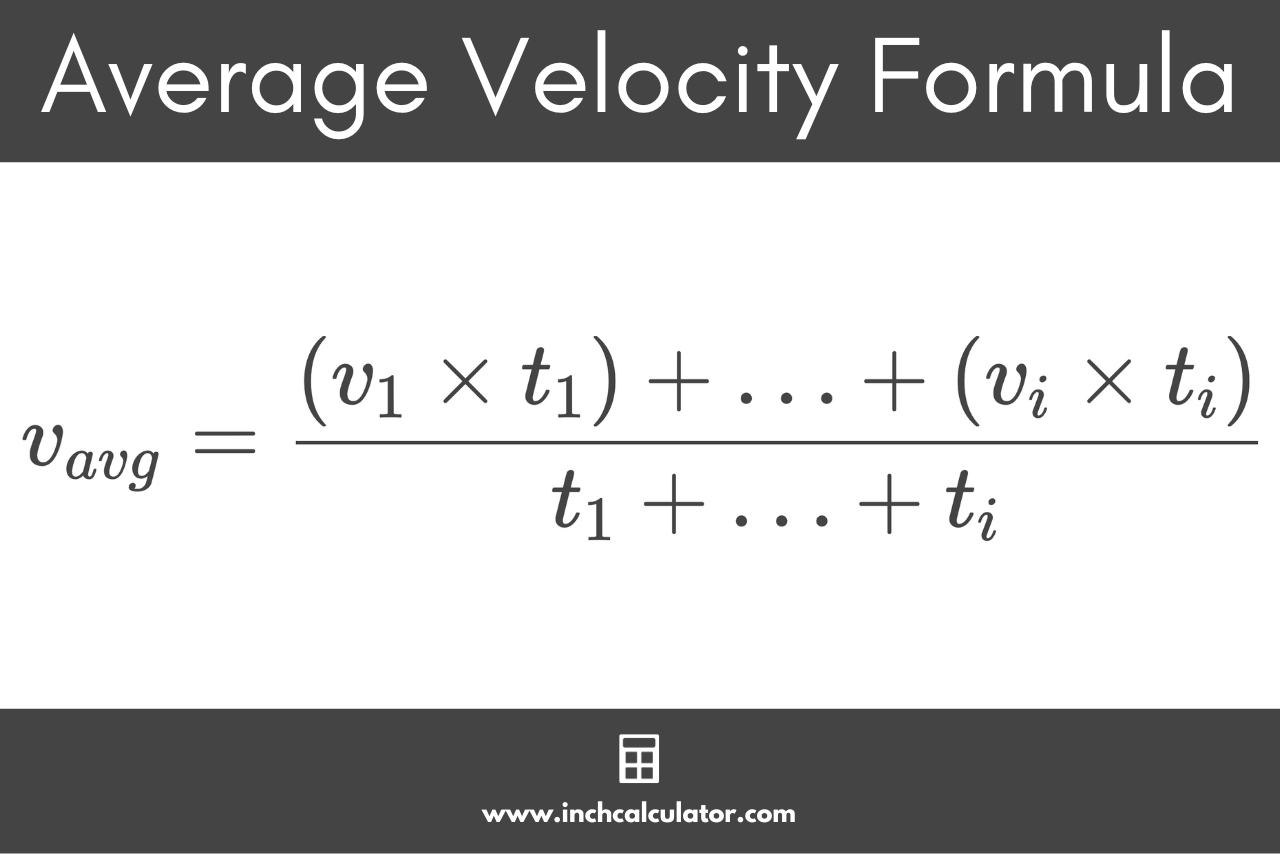# Velocity Calculator

Calculate final velocity, initial velocity, acceleration, or time using the velocity calculator below.

Calculate:

## Final Velocity:

-

### Final Velocity Formula

v_{t}=v_{0}+at
Learn how we calculated this below

## How to Calculate Velocity

Velocity is a fundamental concept in the study of physics, especially in kinematics, the branch of physics that deals with motion. Velocity is the speed of an object in a given direction. It is the rate at which an object moves in a particular direction over time.

There are several methods to calculate velocity, depending on what you know.

### How to Calculate Velocity Using Displacement

The most common approach to calculating velocity is by using displacement. Displacement is the overall change in the position of an object (distance), irrespective of the path taken.

#### Velocity Formula Given Displacement

If an object travels a certain displacement in a given time duration, then you can calculate the velocity using the formula:

v=\frac{s}{t}

The velocity v is equal to the displacement s divided by the time duration t. This is very similar to the method used to calculate speed.It’s important to note that this gives the average velocity over the time period t and not the instantaneous velocity at any given moment.

### How to Calculate Velocity Using Acceleration

Acceleration is the rate of change of velocity with respect to time. If an object starts from a certain initial velocity and is subjected to a constant acceleration, its velocity will change over time.

#### Velocity Formula Given Acceleration

The formula to calculate the final velocity is:

v_{t}=v_{0} + at

The final velocity vt is equal to the initial velocity v0 plus the acceleration a times the duration of time t.You can use this formula for objects undergoing uniform (constant) acceleration.

It’s important to ensure all the units used are consistent. For instance, if velocity is measured in meters per second (m/s) and time in seconds (s), acceleration should be in meters per second squared (m/s²).

### How to Calculate Average Velocity

Average velocity is the total displacement divided by the total time taken. It’s a vector quantity, meaning it has both magnitude and direction. Unlike speed, which only considers the total distance traveled regardless of direction, average velocity takes the direction of motion into account.

#### Average Velocity Formula

The formula to calculate the average velocity is:

v_{avg}=\frac{(v_{1} \times t_{1}) + … + (v_{i} \times t_{i})}{t_{1} + … + t_{i}}

The average velocity vavg is equal to the sum of the products of the velocity vi and time duration ti for each time interval divided by the total time duration.### What's the difference between velocity and speed?

Speed is a scalar quantity that refers only to how fast an object is moving, while velocity is a vector quantity that refers to how fast and in which direction an object is moving. Velocity can have a direction, but speed cannot.

### If acceleration is zero, what happens to velocity?

If acceleration is zero, it means the object is not speeding up or slowing down. Its velocity remains constant. This doesn’t mean the object is at rest unless its velocity is also zero.

### Can velocity be negative?

Yes, velocity can be negative, which indicates that the object is moving in the opposite direction.

### Can an object have a constant speed but a changing velocity?

Yes. If an object is moving in a circular path at a constant speed, its direction (and hence velocity) is constantly changing even though its speed remains the same.